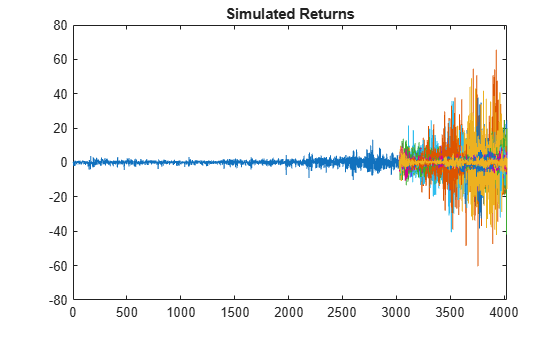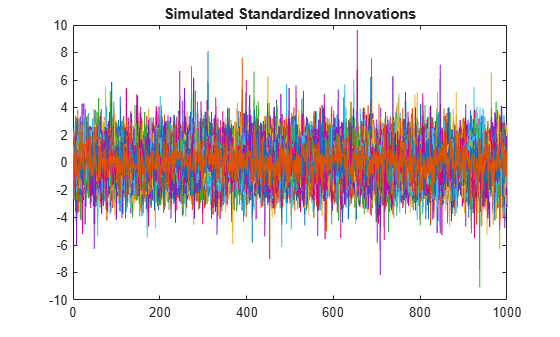Documentation

## Simulate Conditional Mean and Variance Models

This example shows how to simulate responses and conditional variances from a composite conditional mean and variance model.

### Load the Data and Fit a Model

Load the NASDAQ data included with the toolbox. Fit a conditional mean and variance model to the daily returns. Scale the returns to percentage returns for numerical stability

```load Data_EquityIdx nasdaq = DataTable.NASDAQ; r = 100*price2ret(nasdaq); T = length(r); Mdl = arima('ARLags',1,'Variance',garch(1,1),... 'Distribution','t'); EstMdl = estimate(Mdl,r,'Variance0',{'Constant0',0.001});```
``` ARIMA(1,0,0) Model (t Distribution): Value StandardError TStatistic PValue ________ _____________ __________ __________ Constant 0.093488 0.016694 5.6002 2.1414e-08 AR{1} 0.13911 0.018857 7.3771 1.6175e-13 DoF 7.4776 0.88262 8.472 2.4128e-17 GARCH(1,1) Conditional Variance Model (t Distribution): Value StandardError TStatistic PValue ________ _____________ __________ __________ Constant 0.011246 0.0036305 3.0976 0.001951 GARCH{1} 0.90766 0.010516 86.315 0 ARCH{1} 0.089897 0.010835 8.2966 1.071e-16 DoF 7.4776 0.88262 8.472 2.4128e-17 ```
`[e0,v0] = infer(EstMdl,r);`

### Simulate Returns, Innovations, and Conditional Variances

Use `simulate` to generate 100 sample paths for the returns, innovations, and conditional variances for a 1000-period future horizon. Use the observed returns and inferred residuals and conditional variances as presample data.

```rng 'default'; [y,e,v] = simulate(EstMdl,1000,'NumPaths',100,... 'Y0',r,'E0',e0,'V0',v0); figure plot(r) hold on plot(T+1:T+1000,y) xlim([0,T+1000]) title('Simulated Returns') hold off```The simulation shows increased volatility over the forecast horizon.

### Plot Conditional Variances

Plot the inferred and simulated conditional variances.

```figure plot(v0) hold on plot(T+1:T+1000,v) xlim([0,T+1000]) title('Simulated Conditional Variances') hold off```The increased volatility in the simulated returns is due to larger conditional variances over the forecast horizon.

### Plot Standardized Innovations

Standardize the innovations using the square root of the conditional variance process. Plot the standardized innovations over the forecast horizon.

```figure plot(e./sqrt(v)) xlim([0,1000]) title('Simulated Standardized Innovations')```The fitted model assumes the standardized innovations follow a standardized Student's t distribution. Thus, the simulated innovations have more larger values than would be expected from a Gaussian innovation distribution.